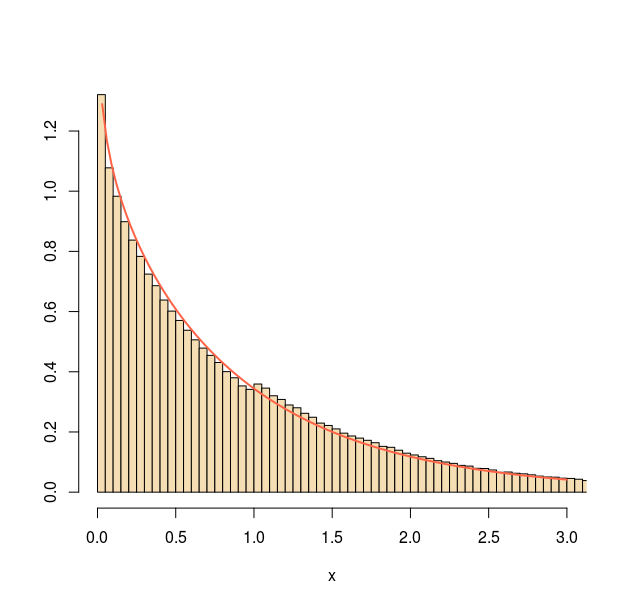Want to share your content on R-bloggers? click here if you have a blog, or here if you don't.When answering an X validated question on running an accept-reject algorithm for the Gamma distribution by using a mixture of Beta and drifted (bt 1) Exponential distributions, I came across the above glitch in the fit of my 10⁷ simulated sample to the target, apparently displaying a wrong proportion of simulations above (or below) one.

```a=.9
g<-function(T){
x=rexp(T)
v=rt(T,1)<0
x=c(1+x[v],exp(-x/a)[!v])
x[runif(T)1)*exp(1-x)+a*(x<1)*x^a/x)*a]}```

It took me a while to spot the issue, namely that the output of

```  z=g(T)
while(sum(!!z)was favouring simulations from the drifted exponential by truncating. Permuting the elements of z before returning solved the issue (as shown below for a=½)!var vglnk = { key: '949efb41171ac6ec1bf7f206d57e90b8' };

(function(d, t) {
var s = d.createElement(t); s.type = 'text/javascript'; s.async = true;